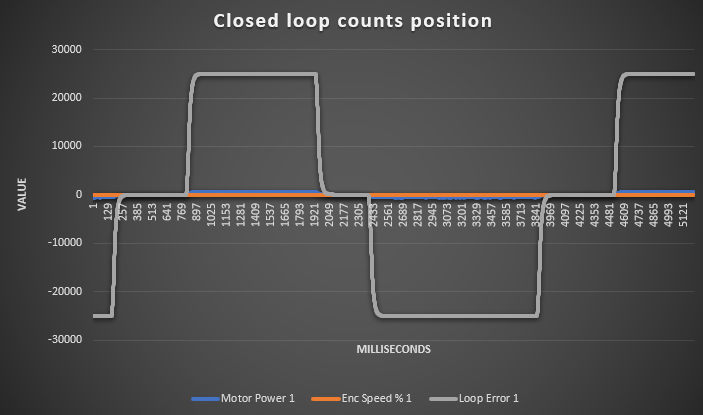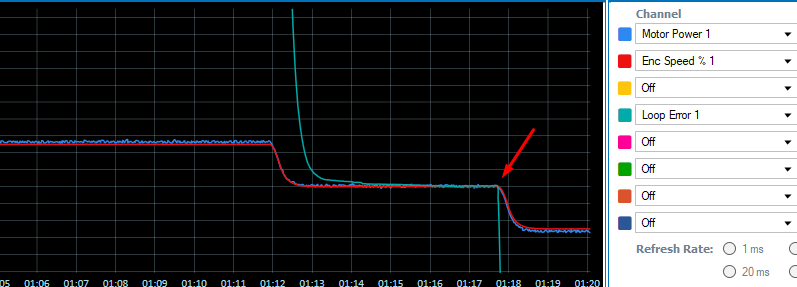In this article, we are going to use a test script which measures the time required for a motor to reach 2 desired positions(measured in encoder counts), in closed loop counts position mode. It also gives the user the ability to configure several parameters like the constant velocity with which it will perform the movement, as well as the acceptable position error threshold, depending on the precision that is required.

Closed loop count position mode, uses the quadrature encoder's counts as feedback for the rotor's position.

Counts = 4 * PPR  for each full turn of the rotor.

If the PID gains set for closed loop position are aggressive, it is very common to face motor and/or power source oscillations. Unlike the closed loop speed mode, the feedback is more sensitive so we propose to test initially with a small P gain only. Increase it until the steady state is reached in a satisfactory time. Then you can slightly decrease P gain and add an integral gain. Then increase it so that steady state error reaches 0.

Note that if the FOC & Torque gains have been set as well, these will be used in cascade with position gains. This means that you should use very low P and I gains. Some times it is necessary to use only P gain to avoid overshoots

Example :

P = 0.01  |     I = 0     |     D = 0   ---> Steady state reached slowly

P = 0.05  |     I = 0       |     D = 0   ---> Steady state reached fast enough

P = 0.03  |     I = 0.01  |     D = 0   ---> Loop error reaches 0 slowly

P = 0.03  |     i = 0.02  |     D = 0   ---> Loop error reaches 0 fast enough

The error_threshold parameter, can be set to a low value to achieve a high precision in the end/start positions.

For example, let's suppose we have a 1024 PPR quadrature encoder and we want 0.5° precision.

counts = 4 * 1024 = 4096 for a 360 ° turn.

So a 0.5°  resolution corresponds to a 4096 * 0.5 / 360  = 5.68 counts => 5 counts. So we shall set the parameter

error_threshold to 5. Keep in mind that a super high resolution(e.g 1 count) may not be achievable due to noise.

The script samples the error 10 times in an interval of 200 milliseconds, then checks if the average error is below that threshold to consider the movement finished.

Here is the graph showing the loop error over time:The motor speed and power are very low in comparison to counts, so let us zoom in to see how the error slowly reaches 0 :The script :

Disclaimer : This script has not been subject to formal tests and validations. It is believed to be fault free but there is always the case that something does not work as expected. It is the user's responsibility to use this script under a safe environment

``'Disclaimer : This script has not been subject to formal tests and validations. It is believed to be fault free but there is always the case that something does not work as expected. ``
``'It is the user's responsibility to use this script under a safe environment``

'Script to calculate the time it takes to reach desired position on Closed loop counts position mode

'with a configurable precision measured in encoder counts (error_threshold parameter)

'This mode uses the quadrature encoder counts (4 * PPR for a full turn) as feedback.

'The command for movement is !P [ch] [counts]

'Channel 1 is used

'Keep in mind that any electrical noise in the sensor's wires might affect the feedback's resolution and make a low error threshold unachievable.

option explicit

'########################## Parameters #################################

'change the following parameters depending on the range of movement you want, the speed(RPM), as well as the error threshold which is considered ‘acceptable, below which the movement is considered successful.

#define positive_end 1000000 'quadrature encoder counts to max position

#define negative_end -1000000 'quadrature encoder counts to min position

#define constant_speed 1000 '(RPM)

#define error_threshold 8  'error = (desired counts - current counts). Below error_threshold value, the position is considered reached successfully (minimum value=1)

dim time as integer

dim as integer

dim average as integer

time

setConfig(_MVEL constant_speed)

gosub run_negative

wait(1000)

'####################### Main Test : #######################################

main

time

gosub run_positive

print("Time to reach positive end = " time"\n"

wait(1000)

gosub run_negative

print("Time to reach negative end = " time"\n")

wait(1000)

goto main

'##########################  Subroutines : #######################################

run_negative:

setCommand(_P negative_end'GO to initial position before the test

wait(100)

time 100

while abs(getvalue(_SR 1) > 10'wait for the speed to drop before sampling loop error

setCommand(_P negative_end

wait(1)

time+=

End While

wait(200'wait for the error to cross 0

time += 200

gosub error_correction_negative

return

run_positive:

setCommand(_P positive_end'GO to initial position before the test

wait(100)

time 100

while abs(getvalue(_SR 1) > 10'wait for the speed to drop before sampling loop error

setCommand(_P positive_end

wait(1)

time+= 1

End While

wait(200'wait for the error to cross 0

time += 200

gosub error_correction_positive

return

error_correction_negative:

average =  abs(getValue(_E 1))

while average error_threshold

average

for i=andwhile i<10

average += abs(getValue(_E 1))

wait(20)

time += 20

next

average average 10

end while

return

error_correction_positive:

average =  abs(getValue(_E 1))

while average error_threshold

average

for i=andwhile i<10

average += abs(getValue(_E 1))

wait(20)

time += 20

next

average average 10

end while

return